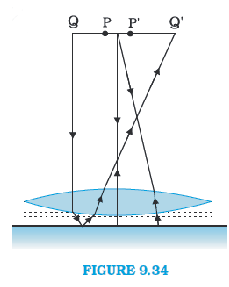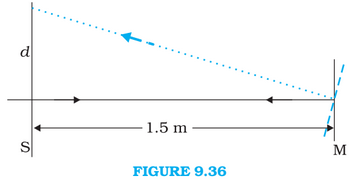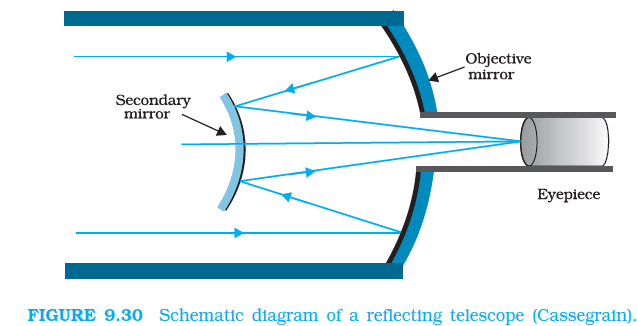## Filters

Sort by :
Clear All
Q

Q 9.25  What should be the distance between the object in figure 9.23 and the magnifying glass if the virtual image of each square in the figure is to have an area of 6.25 mm2. Would you be able to see the squares distinctly with your eyes very close to the magnifier?

Given Virtual image area = 6.25 mm2 Actual ara = 1 mm2 We can calculate linear magnification as  we also know  Now, according to the lens formula  and  Since the image is forming at a distance which is less than 25 cm, it can not be seen by eye distinctively.

Q 9.24  (a)  ) At what distance should the lens be held from the figure in Exercise 9.29 in order to view the squares distinctly with the maximum possible magnifying power?

(b) What is the magnification in this case?

(c) Is the magnification equal to the magnifying power in this case?

a) maximum magnifying is possible when our image distance will be equal to minimum vision point that is,      (Given) Now according to the lens formula Hence required object distance for viewing squares distinctly is 7.14 cm away from the lens. b) Magnification of the lens:   c) Magnifying power    Since the image is forming at near point ( d = 25 cm ), both magnifying power and...

Q 9.32  Figure 9.34 shows an equiconvex lens (of refractive index 1.50) in contact with a liquid layer on top of a   plane mirror. A small needle with its tip on the principal axis is moved along the axis until its inverted image is found at the position of the needle. The distance of the needle from the lens is measured to be 45.0cm. The liquid is removed and the experiment is repeated. The new distance is measured to be 30.0cm. What is the refractive index of the liquid?Given The focal length of the convex lens  here liquid is acting like the mirror so, the focal length of the liquid  the focal length of the system(convex + liquid)  Equivalent focal length when two optical systems are in contact   Now, let us assume refractive index of the lens be  The radius of curvature are  and . As we know, Now, let refractive index of liquid be  The radius of...

Q 9.31 Light incident normally on a plane mirror attached to a galvanometer coil retraces backwards as shown in Fig. 9.33. A current in the coil produces a deflection of 3.5o of the mirror. What is the displacement of the reflected spot of light on a screen placed 1.5 m away?Given Angle of deflection  The distance of the screen from the mirror  The reflected rays will bet deflected by twice angle of deviation that is   Now from the figure, it can be seen that Hence displacement of the reflected spot of the light is .

Q9.30  A Cassegrain telescope uses two mirrors as shown in Fig. 9.30. Such a telescope is built with the mirrors 20mm apart. If the radius of curvature of the large mirror is 220mm and the small mirror is 140mm, where will the final image of an object at infinity be?Given, Distance between the objective mirror and secondary mirror  The radius of curvature of the Objective Mirror    So the focal length of the objective mirror   The radius of curvature of the secondary mirror    so, the focal length of the secondary mirror   The image of an object which is placed at infinity, in the objective mirror, will behave like a virtual object for the secondary...

Q 9.29  (c) What is the height of the final image of the tower if it is formed at 25cm

Given, image is formed at a distance  = 25cm As we know, magnification of eyepiece lens is given by : Now,  Height of the final image is given by : Therefore, the height of the final image will be 28.2 cm

Q 9.29  (b) If this telescope is used to view a 100 m tall tower 3 km away, what is the height of the image of the tower formed by the objective lens?

Given, focal length of the objectove lens = = 140cm focal length of the eyepiece lens =  = 5 cm Height of tower  = 100m Distance of object which is acting like a object  = 3km = 3000m. The angle subtended by the tower at the telescope  Now, let the height of the image of the tower by the objective lens is  . angle made by the image by the objective lens  : Since both, the angles are the same...

Q 9.29 (a)  For the telescope described in Exercise 9.28 (a), what is the separation between the objective lens and the eyepiece?

a) Given, focal length of the objective lens = = 140cm focal length of the eyepiece lens =  = 5 cm  The separation between the objective lens and eyepiece lens is given by: Hence, under normal adjustment separation between two lenses of the telescope is 145 cm.

Q 9.28  A small telescope has an objective lens of focal length 140cm and an eyepiece of focal length 5.0cm. What is the magnifying power of the telescope for viewing distant objects when

(b) the final image is formed at the least distance of distinct vision (25cm)?

Given, the focal length of the objective lens  the focal length of the eyepiece lens  normally, least distance of vision = 25cm Now, as we know magnifying power when the image is at d = 25 cm is Hence magnification, in this case, is 33.6.

Q 9.28  A small telescope has an objective lens of focal length 140cm and an eyepiece of focal length 5.0cm. What  is the magnifying power of the telescope for viewing distant objects when
(a) the telescope is in normal adjustment (i.e., when the final image is at infinity)?

Given, the focal length of the objective lens  the focal length of the eyepiece lens  normally, least distance of vision = 25cm Now, As we know magnifying power:   Hence magnifying power is 28.

Q9.27  An angular magnification (magnifying power) of 30X is desired using an objective of focal length 1.25cm and an eyepiece of focal length 5cm. How will you set up the compound microscope?

Given, magnifying power = 30 objective lens focal length = 1.25cm eyepiece lens focal length = 5 cm Normally, image is formed at distance d = 25cm Now, by the formula; Angular magnification by eyepiece:    From here, magnification by the objective lens :      since   ( ) According to the lens formula: from here, hence object must be 1.5 cm away from the objective lens. Now for the...

Q 9.26  (e) When viewing through a compound microscope, our eyes should be positioned not on the eyepiece but a short distance away from it for best viewing. Why? How much should be that short distance between the eye and eyepiece?

When we view through a compound microscope, our eyes should be positioned a short distance away from the eyepiece lens for seeing a clearer image. The image of the objective lens in the eyepiece lens is the position for best viewing. It is also called "eye-ring" and all reflected rays from lens pass through it which makes it the ideal position for the eye for the best view. When we put our eyes...

(d)  Why must both the objective and the eyepiece of a compound microscope have short focal lengths?

We need more magnifying power and angular magnifying power in a microscope in order to use it effectively. Keeping both objective focal length and eyepiece focal length small makes the magnifying power greater and more effective.

Q 9.26  (c)  Magnifying power of a simple microscope is inversely proportional to the focal length of the lens. What then stops us from using a convex lens of smaller and smaller focal length and achieving greater and greater magnifying power?

Firstly, grinding a lens with very small focal length is not easy and secondly and more importantly, when we reduce the focal length of a lens, spherical and chronic aberration becomes more noticeable. they both are defects of the image, resulting from the ways of rays of light.

Q 9.26.   (b) In viewing through a magnifying glass, one usually positions one’s eyes very close to the lens. Does angular magnification change if the eye is moved back?

Yes, angular magnification will change if we move our eye away from the lens. this is because then angle subtended by lens would be different than the angle subtended by eye. When we move our eye form lens, angular magnification decreases. Also, one more important point here is that object distance does not have any effect on angular magnification.

Q 9.26   (a)  The angle subtended at the eye by an object is equal to the angle subtended at the eye by the virtual image produced by a magnifying glass. In what sense then does a magnifying glass provide angular magnification?

Angular magnification is the ratio of tangents of the angle formed by object and image from the centre point of the lens. In this question angle formed by the object and a virtual image is same but it provides magnification in a way that, whenever we have object place before 25cm, the lens magnifies it and make it in the vision range. By using magnification we can put the object closer to the...

Q 9.23  A card sheet divided into squares each of size 1 mm2 is being viewed at a distance of 9 cm through a magnifying glass (a converging lens of focal length 10 cm) held close to the eye.

(a) What is the magnification produced by the lens? How much is the area of each square in the virtual  image?

(b) What is the angular magnification (magnifying power) of the lens?

(c) Is the magnification in (a) equal to the magnifying power in (b)? Explain.

Given, Object distance u = -9cm Focal length of convex lens = 10cm According to the lens formula a) Magnification The area of each square in the virtual image b) Magnifying power c) No,  . Both the quantities will be equal only when image is located at the near point |v| = 25 cm

Q 9.21 (b) An object 1.5 cm in size is placed on the side of the convex lens in the arrangement (a) above. The distance between the object and the convex lens is 40cm. Determine the magnification produced by the two-lens system, and the size of the image.

Given  Object height = 1.5 cm  Object distance from convex lens = -40cm According to lens formula Magnificatio due to convex lens:   The image of convex lens will act as an object for concave lens, so, Magnification due to concave lens : The combined magnification:   Hence height of the image =   = 0.652 * 1.5 = 0.98cm Hence height of image is 0.98cm.

Q 9.22  At what angle should a ray of light be incident on the face of a prism of refracting angle so that it just suffers total internal reflection at the other face? The refractive index of the material of the prism is .

Let prism be ABC ,   as emergent angle ,    (approx) Now as we know in the prism  Hence,  Now applying snells law at surface AB Hence the angle of incident is 29.75 degree.

Q 9.21 (a)   Determine the ‘effective focal length’ of the combination of the two lenses in Exercise 9.10, if they are placed 8.0cm apart with their principal axes coincident. Does the answer depend on which side of the combination a beam of parallel light is incident? Is the notion of effective focal length of this system useful at all?

Here there are two cases, first one is the one when we see it from convex side i.e. Light are coming form infinite and going into convex lens first and then goes to concave lens afterwords. The second case is a just reverse of the first case i.e. light rays are going in concave first. 1)When light is incident on convex lens first Now this will act as an object for the concave...
Exams
Articles
Questions